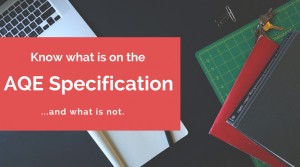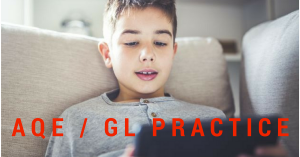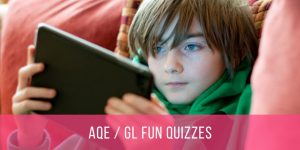# AQE SpecificationAccording to the AQE website, the specification below covers the details of what will be and what will not be tested (which can be equally important) on the AQE test.

## English

Questions will assess the AT2 Reading and AT3 Writing sections of the Programme of Study for English. The level of difficulty of the questions will not exceed that indicated by the level descriptions for Level 5.

## Mathematics

Questions set will relate to the Key Stage 2 Programme of Study for mathematics. They will not assume a knowledge or understanding beyond this. The level of difficulty of the questions will not exceed that indicated by the level descriptions for Level 5.

Questions will address content detailed in the Attainment Targets: Number; Measures; Shape and Space; and Handling Data, as set out in the extracts from the Programme of Study reproduced below. Where material has been omitted or where limitations have been put on specific content for the purposes of the tests, these have been indicated in bold text immediately following the particular section of the Programme of Study to which they relate.

The nature of the Common Entrance Assessment is such that aspects of the Programme of Study that involve certain types of activity, such as discussion or practical activities, will not be included. The use of the calculator will not be permitted in the tests.

As Processes in Mathematics pervades the entire mathematics programme, aspects of the strands of Using Mathematics, Communicating Mathematically, and Mathematical Reasoning will be included in questions, where appropriate. While the use of the calculator is not permitted in the tests, questions relating to the interpretation of a calculator display may be included.

### NUMBER

Understanding Number and Number Notation
Pupils should have opportunities to:

1. Read, write and order whole numbers, initially to 100 and progressing to using any whole number, understanding that the position of a digit signifies its value; use their understanding of place value to develop computational methods;
2. Extend understanding of place value to include decimals, initially to one decimal place and then up to two decimal places; use this to multiply and divide numbers by 10, 100, and 1000;
3. Estimate within calculations, initially with numbers within 100 and extending to all whole numbers; approximate numbers to the nearest 10 or 100; estimate and approximate to gain a feeling for the size of a solution to a problem, for example, understand that 32 × 9 is approximately 30 × 10;
4. Understand and use, in context, vulgar fractions, decimal fractions and percentages; understand the equivalence of simple fractions; explore the relationships between fractions and percentages, for example, understand that half price is the same as 50% off.
Relationships other than those listed below will not be included in the tests:
½ = 0.5 = 50%
¼ = 0.25 = 25% ¾ = 0.75 = 75%
1 = 1.00 = 100%
1/10 = 0.1 = 10%
⅓ = 0.33 = 33⅓%

Patterns, Relationships and Sequences
Pupils should have opportunities to:

1. Explore and predict patterns and sequences of whole numbers initially within 100 and extending to larger numbers, including counting in different sizes of step, doubling and halving numbers, finding multiplication patterns in the hundred square, predicting subsequent numbers in a sequence; follow simple sets of instructions to generate a sequence;
Questions will not be set on devising rules for determining sequences
2. Understand and use multiples and factors and terms including prime, square and cube; appreciate that multiplication and division are inverse operations;
3. Interpret, generalise and use simple relationships expressed in numerical, spatial and practical situations, for example, finding equivalent forms of two digit numbers; understanding square and triangular numbers; understand and use simple function machines;
4. Understand that a letter can stand for an unknown number, for example, 6 + a = 24.
Questions will only involve the use of whole numbers and a letter will not be squared or cubed.

Operations and their Applications
Pupils should have opportunities to:

1. Consolidate knowledge of addition and subtraction facts to 20; understand and use this knowledge to calculate quickly facts that they cannot recall; add mentally two two-digit numbers up to 100 and subtract mentally one two-digit number from another; know the multiplication facts to 10 × 10; use these facts when solving problems;
2. Engage in a range of activities to develop understanding of the four operations of number and their interrelationships;
Questions will not be set on appreciation of the use of brackets
3. Develop a range of non-calculator methods of computation to include addition and subtraction with up to two decimal places and multiplication and division of decimals by whole numbers; use these operations to solve problems, using a calculator where necessary.

Money
Pupils should have opportunities to:

1. Understand and use the conventional way of recording money; use the four operations to solve problems;
2. Estimate and approximate to gain a feeling for the size of a solution to a problem before carrying out a calculation;
3. Interpret a calculator display in relation to money.### MEASURES

Pupils should have opportunities to:

1. Develop skills in estimation of length, weight, volume/capacity, time, area and temperature through practical activities, using metric units where appropriate;
2. Develop the language associated with a wider range of metric units and be confident with the terms metre, gram and litre, and their relevant prefixes of kilo, centi, milli;
3. Appreciate important ideas about measurement including the continuous nature of measurement and the need for appropriate accuracy;
4. Choose and use appropriate metric units and measuring instruments in a variety of situations, interpreting numbers on a range of measuring instruments, for example, measure the perimeter of the playground to the nearest metre using a trundle wheel;
5. Understand the relationship between units, for example, know that kilograms and grams are used to weigh food; convert from one metric unit to another, for example, know that 175 centimetres is 1.75 metres; use the four operations to solve problems, working with up to three decimal places, where appropriate;
Pupils will not be expected in the test to know the Imperial units still in common use.
6. Understand and use negative numbers in context, for example, know that if the temperature rises during the day from -3˚C to 4˚C, the temperature has risen by 7 degrees;
7. Understand the concept of perimeter and calculate the perimeter of simple shapes; find areas by counting squares and volumes by counting cubes; calculate areas and volumes of simple shapes in two and three dimensions;
8. Understand and use scale in the context of simple maps and drawings, for example: draw a simple plan of the classroom and know that one centimetre square represents one square metre; calculate the actual distance as the crow flies between two places on a map using the scale of 1cm to 1 km;
Questions will be limited to calculating scale from simple given drawings
9. Know the units of measurement of time and the relationship between them;
10. Recognise times on the analogue clock, including the hour, half and quarter hours, five minute intervals and one minute intervals; understand the relationship between the twelve and twenty-four hour clocks, including am and pm; read analogue and digital displays and understand the relationship between them; use timetables involving the twenty-four hour clock and perform simple calculations related to the timetables;
11. Know the months of the year; explore calendar patterns.### SHAPE & SPACE

Exploration Of Shape

Pupils should have opportunities to:

1. Use materials, for example, geoboards, construction sets and paper, to construct and handle a wide range of regular and irregular 2-D shapes; classify these through examination of angles and sides; look for line symmetries in practical situations;
Questions will not be set on recognition of rotational symmetry
2. Reflect shapes, for example, by using a mirror; use shapes to explore and create tessellations; name and describe common 2-D shapes including squares, rectangles, circles, triangles, hexagons and pentagons;
Questions will not be set on the meaning of congruence in 2-D shapes
3. Use materials, for example, blocks, construction sets and cereal packets, to construct various 3-D shapes; investigate the number of faces, edges and vertices on these shapes; name and describe common 3-D shapes including cubes, cuboids, cones, cylinders, spheres, triangular prisms and pyramids; use nets to explore the relationship between 2-D and 3-D shapes;
4. Recognise geometrical properties and use these to solve problems;
Questions will relate only to the internal angles of triangles and quadrilaterals.

Position, Movement and Direction

Pupils should have opportunities to:

1. Investigate practically ¼ turns, ½ turns and whole turns to understand the notion of angle in the context of turning; find right angles in 2-D and 3-D shapes in the environment; understand clockwise and anticlockwise; know the eight points of the compass; use a programmable device, for example, a Roamer, to extend understanding of movement and turning;
2. Develop the language associated with line and angle, including vertical, horizontal, perpendicular, parallel, acute, obtuse and reflex;
3. Recognise properties of acute, obtuse and reflex angles, for example, know that an acute angle is less than a right angle and that a reflex angle is greater than two right angles;
4. Investigate angles in triangles, including scalene, right-angled, equilateral and isosceles, and quadrilaterals including square, rectangle, rhombus, kite, parallelogram and trapezium;
Pupils will not be required in the test to measure or to draw angles
5. Use co-ordinates to plot points and draw shapes in the first quadrant;
6. Be introduced to a programming language, for example, LOGO, and use it to create pictures and patterns and to generate mathematical shapes.

Collect, Represent and Interpret Data

Pupils should have opportunities to:

1. Use data drawn from a range of meaningful situations;
2. Collect, classify, record, represent and interpret discrete numerical data, using graphs, tables and diagrams, including Venn, decision tree and Carroll diagrams, pictograms, block graphs, bar charts, bar-line graphs and line graphs with the axis starting at zero (initially with given intervals), explain their work orally or through writing, and draw conclusions;
3. Interpret tables and lists used in everyday life, for example, those found in a catalogue or road safety accident report; interpret a wide range of graphs and diagrams including a pie chart; create and interpret frequency tables, including those for grouped discrete data, for example, birthdays; use tallying methods, including the 5-bar gate;
4. Design an observation sheet and use it to record a set of data leading to a frequency table; collate and analyse the results; progress to designing and using a data collection sheet, interpreting the results;
Questions will not be set relating to the use of computer packages to produce graphical representations of data
5. Understand, calculate and use the mean and range of a set of discrete data, for example, calculating the mean score of two teams that have played different numbers of games in order to compare their performance.

Introduction to Probability

Pupils should have opportunities to:

1. Become familiar with and use the language of probability including certain, uncertain, likely, unlikely, impossible and fair, by participating in games and other practical activities;
2. Understand possible outcomes of simple random events, for example, that buttered toast will fall with either the buttered side up or the buttered side down; understand that there is a degree of uncertainty about the outcome of some events, while others are certain or impossible, for example, it is: certain to get dark tonight; impossible for a person to turn into a fish; uncertain whether or not it will rain tomorrow;
3. Place events in order of “likelihood”; understand and use the idea of “fifty-fifty” or “evens” and know whether events are more or less likely than this, for example, know that if a die is thrown there is an equal chance of an odd or even number but the chance of getting a 5 is less than an even chance.### More about The Transfer Tutor (Multi-Award Winning App)

The Transfer Tutor App is an online site dedicated to providing fun and interactive quizzes aligned directly to the AQE / GL specifications.  All quizzes are broken down into manageable topics and can be done on any mobile or tablet device, so it’s an great way for kids to learn and prepare for the test.

If your child is in P6 and you want the licence that will last you until December 2019, simply click on the picture below to buy the Penguin Licence at only £45 for the whole year.

### (Expires December 2019)

However, if there are younger siblings, you can choose a longer licence, for only another £15 per additional years! So, if this is something you would like to explore, click here to get more details and to look at the other membership options.

To give you a feel for the quizzes, you can start a free one by clicking on the button below.

The quizzes have been a great success with parents and kids and received many, raving 5* Facebook Reviews in the last year, so definitely worth a look and read how so many people have loved using the app.